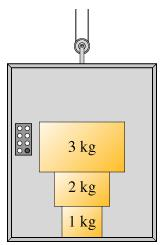# Problem: Assume the elevator is moving upward at increasing speed. Rank the magnitude of the forces.Rank from largest to smallest. To rank items as equivalent, overlap them.Answer the following questions with reference to the eight forces defined as follows.the force of the 3kg block on the 2kg block, F3 on 2the force of the 2kg block on the 3kg block, F2 on 3the force of the 3kg block on the 1kg block, F3 on 1the force of the 1kg block on the 3kg block, F1 on 3the force of the 2kg block on the 1kg block, F2 on 1the force of the 1kg block on the 2kg block, F1 on 2force of the 1kg block on the floor, F1 on floorforce of the floor on the 1kg block, Ffloor on 1

###### FREE Expert Solution

We're finding the magnitude of the forces.

98% (345 ratings)###### Problem DetailsAssume the elevator is moving upward at increasing speed. Rank the magnitude of the forces.

Rank from largest to smallest. To rank items as equivalent, overlap them.

Answer the following questions with reference to the eight forces defined as follows.

the force of the 3kg block on the 2kg block, F3 on 2
the force of the 2kg block on the 3kg block, F2 on 3
the force of the 3kg block on the 1kg block, F3 on 1
the force of the 1kg block on the 3kg block, F1 on 3
the force of the 2kg block on the 1kg block, F2 on 1
the force of the 1kg block on the 2kg block, F1 on 2
force of the 1kg block on the floor, F1 on floor
force of the floor on the 1kg block, Ffloor on 1